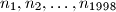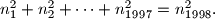### Junior Balkan MO 1997

Kvaliteta:
Avg: 2.5
Težina:
Avg: 3.3
Let$n_1, n_2, \ldots, n_{1998}$ be positive integers such that$n_1^2 + n_2^2 + \cdots + n_{1997}^2 = n_{1998}^2.$ Show that at least two of the numbers are even.